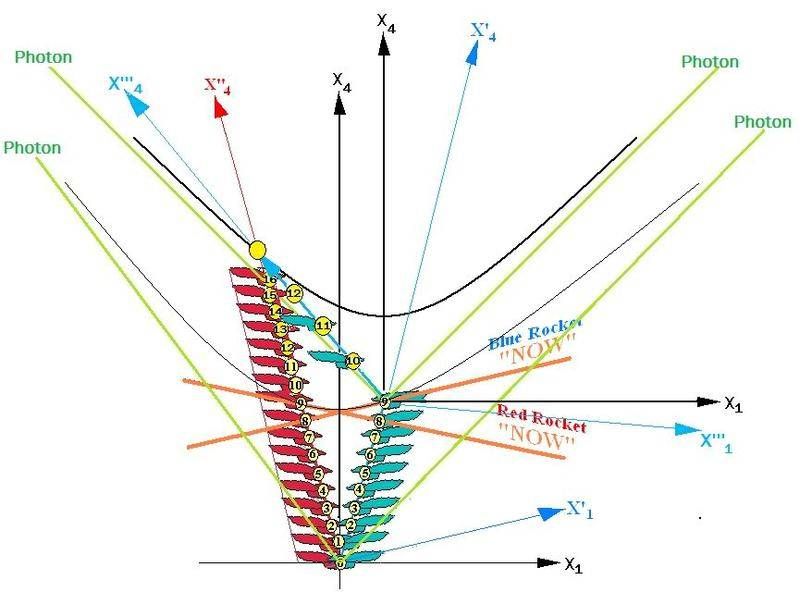# Visualizing Minkowski geometry

Is there a better way of visualizing (flat) spacetime than Minkowski diagrams? In Minkowski diagrams, the distance between events doesn't match the Minkowski norm, and I feel that it may be possible to change this with some other representation of spacetime, maybe involving curved surfaces or something.

A.T.
Is there a better way of visualizing (flat) spacetime than Minkowski diagrams? In Minkowski diagrams, the distance between events doesn't match the Minkowski norm, and I feel that it may be possible to change this with some other representation of spacetime, maybe involving curved surfaces or something.
This is tricky. A geometric distance in a diagram is always a real positive value, but the Minkowski norm can get imaginary. Even if you restrict yourself to the time-like part, you have the problem that the distance between all points on the light cone is 0, so this would be a singularity in your diagram.

There is an alternative called Epstein diagrams, where the temporal axis represents proper time, and the distance (path interval) represents coordiante time. So you have both times represented as lengths directly in the diagram:

Here is a comparison of both diagram types for the standard twins scenario:.

Hmm, well would it be possible to at least represent a portion of the light cone?

Is there a better way of visualizing (flat) spacetime than Minkowski diagrams? In Minkowski diagrams, the distance between events doesn't match the Minkowski norm, and I feel that it may be possible to change this with some other representation of spacetime, maybe involving curved surfaces or something.

You can get half way there by beginning with a symmetric space-time diagram. You can arbitrarily select a rest frame for which each of the twins is moving at the same speed in opposite directions (even if you must depict the entire earth as moving with the twin remaining at home on the earth).

In the sketch below the distances for the red guy and blue guy are the same for the outgoing trip (you don't have to worry about hyperbolic calibration curves). So, when each of the twins is at their respective station number 9 in the diagram, we have the basic time dilation situation where red thinks blue's clock runs slow (he sees blue at blue's station 8) and blue thinks red's clock is running slow (he sees red at red's station 8). We have established a "NOW" for red and a "NOW" for blue at each station 9 (same elapsed proper time for each). The respective X1 dimensions at that instant represent the instantaneous 3-D worlds that each observer lives in (they literally live in different 3-D worlds).

But, then the blue guy has to change to a new inertial frame so he can take the short cut to catch up with the red guy. So, for the return trip you won't get the diagram you were looking for. Now, you have to bring in a new hyperbolic calibration curve for blue's new coordinates. So, you see blue's stations 10, 11, 12, etc. spaced out on the screen as compared to red's. Clearly the blue guy has not aged as much as red by the time they meet up again.Last edited:
Is there a better way of visualizing (flat) spacetime than Minkowski diagrams? In Minkowski diagrams, the distance between events doesn't match the Minkowski norm, and I feel that it may be possible to change this with some other representation of spacetime, maybe involving curved surfaces or something.

The invariants in Minkowski diagrams with xt and x't' coordinate systems are the areas, rather than lengths.

robphy
Homework Helper
Gold Member
The invariants in Minkowski diagrams with xt and x't' coordinate systems are the areas, rather than lengths.

Yes, indeed.
This is a feature of the diagrams in my paper: